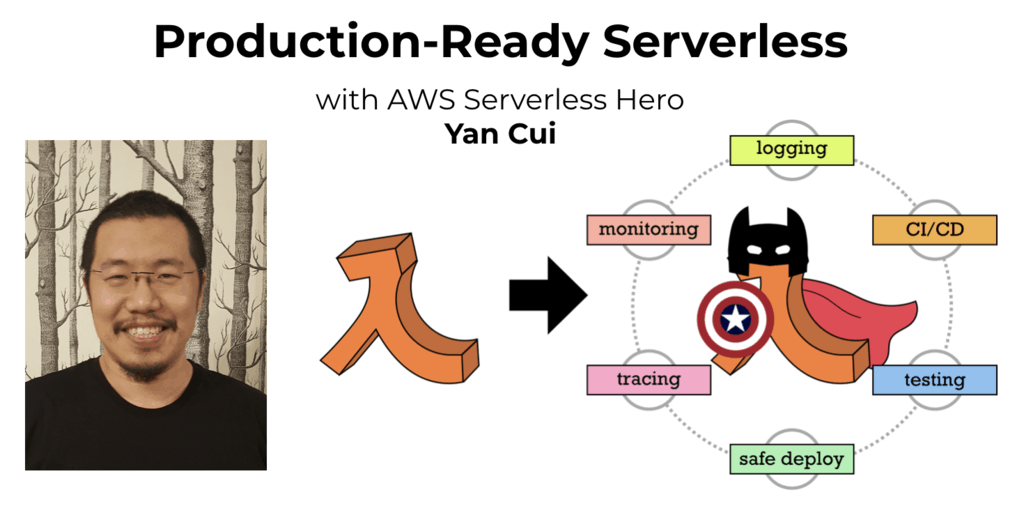# Project Euler – Problem 44 Solution

Check out my new course Learn you some Lambda best practice for great good! and learn the best practices for performance, cost, security, resilience, observability and scalability.

#### Problem

Pentagonal numbers are generated by the formula, Pn=n(3n-1)/2. The first ten pentagonal numbers are:

1, 5, 12, 22, 35, 51, 70, 92, 117, 145, …

It can be seen that P4 + P7 = 22 + 70 = 92 = P8. However, their difference, 70 – 22 = 48, is not pentagonal.

Find the pair of pentagonal numbers, Pj and Pk, for which their sum and difference is pentagonal and D = |Pk – Pj| is minimised; what is the value of D?

#### Solution

```open System.Collections.Generic

// define the Pentagonal function P
let P n = n*(3*n-1)/2

// use a dictionary as cache
let mutable cache = new Dictionary<int, int>()
[1..5000] |> List.iter (fun n -> cache.[n] <- P n)

// function to check if a number is pentagonal by looking at the cached values
let isPentagonal n = cache.ContainsValue n

// predicate function to check if Pk and Pj's sum and diff are both pentagonal
let predicate (k, j) =
let pk = cache.&#91;k&#93;
let pj = cache.&#91;j&#93;
isPentagonal (pj + pk) && isPentagonal (pk - pj)

// the sequence of k, j pairs to check
let kjSeq =
&#91;1..5000&#93;
|> List.collect (fun k -> [1..k-1] |> List.rev |> List.map (fun j -> (k, j)))

// get the first pair of k, j whose sum and difference are both pentagonal
let (k, j) = kjSeq |> Seq.filter predicate |> Seq.head

let answer = (P k) - (P j)
```

Having experimented with a few alternative implementations which did not use a mutable dictionary and waited impatiently for the code to never return I settled on this solution which caches the first 5000 values in the pentagonal number sequence for quick lookup later on in the solution.

Going into more detail about the solution itself, to ensure |Pk – Pj| is minimised, the value of k and the difference between k and j must be minimised too as the further apart Pk and Pj are in the pentagonal sequence the bigger their difference will be and the bigger k is the bigger Pk will be too.

The rest of the algorithm here is simple, for each value k (between 1 and 5000, being the size of the cache) check for each j where 1 <= j < k, starting from the biggest j, if the sum and difference of Pk and Pj are both pentagonal. The first k and j which satisfy this predicate will have the smallest difference between Pk and Pj.Hi, I’m Yan. I’m an AWS Serverless Hero and the author of Production-Ready Serverless.

I specialise in rapidly transitioning teams to serverless and building production-ready services on AWS.

Are you struggling with serverless or need guidance on best practices? Do you want someone to review your architecture and help you avoid costly mistakes down the line? Whatever the case, I’m here to help.Check out my new course, Learn you some Lambda best practice for great good! In this course, you will learn best practices for working with AWS Lambda in terms of performance, cost, security, scalability, resilience and observability. Enrol now and enjoy a special preorder price of £9.99 (~\$13).Are you working with Serverless and looking for expert training to level-up your skills? Or are you looking for a solid foundation to start from? Look no further, register for my Production-Ready Serverless workshop to learn how to build production-grade Serverless applications!